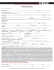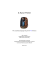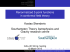# practice exam

## Transcription

practice exam
```Math 425
April 2015
Robin Young
Practice Final Exam
Name:
1. Show that
Z `Z
t
Z
0
0
`
(` − x) f (x) dx.
f (x) dx dt =
0
2. The following system arises in the study of gases:
u − v = (h − α) e−s
w − u = (k − β) e−t
α es = β et .
(a) Under what circumstances can we solve for α, β and u in terms of the remaining
variables?
∂
∂
∂
(b) Calculate ∂s
u, ∂s
α and ∂s
β by exhibiting a linear set of equations for them. No
need to solve the system!
3. Calculate the volume and surface area of the unit sphere.
4. Recall that the work done by the force F along the curve Γ is
Z
Z
W =
F · dx =
F · T ds.
Γ
Γ
2
Use Newton’s law F = m a = m ddt2x to show that the work done is the change of kinetic
energy,
1
1
W = m v2 − m v02 .
2
2
5. Calculate
Z
x dy dz + y dx dz − y dx dy,
S
where S is the boundary of the solid cylinder
x2 + y 2 ≤ 1,
0 ≤ z ≤ 1.
6. (a) Show that the 1-form
ω = (3x2 y 4 − 2x) dx + (4x3 y 3 + 3y 2 ) dy + cosh z dz
is closed.
(b) By integrating along an appropriate curve, show that ω is exact: that is, find a
function (0-form) α such that ω = dα.
7. If F(x, y, z) is a vector field which is differentiable everywhere, show that
Z Z
(∇ × F) · dS
S
vanishes for every closed surface S (i.e. S encloses some volume).
8. Let r = (x1 , . . . , xn ) ∈ Rn and let r = krk be the radial distance function.
2
(a) Calculate the quantities ∇ r and ∇ · r.
(b) If f˜(x1 , . . . , xn ) is a radial function, f˜(x1 , . . . , xn ) = f (r) for a scalar function f ,
show that its Laplacian ∆f˜ ≡ ∇ · ∇ f˜ is given by
∆f˜ = f 00 (r) +
n−1 0
f (r).
r
9. Under what circumstances can the pair of equations
x2 − y 2 − u3 + v 2 = −4
2
2
2xy + y − 2u + 3v
4
= −8
be solved to give (u, v) in terms of (x, y)?
∂
u at the point (x, y, u, v) = (2, −1, 2, 1).
Find ∂x
10. A 1-form ω is radial if it is of the form
ω = f (r) (x dx + y dy + z dz) ,
p
where f is a scalar function and r = x2 + y 2 + z 2 .
(a) Show that a radial 1-form satisfies ω =
1
2
f (r) d(r2 ).
(b) Show that if ω is radial, then its exterior derivative vanishes, d ω = 0.
(c) Find some function g which is a scalar potential for ω, i.e. for which
ω = dg.
3
(1)
(2)
```

### Kenda - Bearclaw HTR - Modern Tire Company### A direct estimate of the radial growth of stellar discs THEORY OBSERVATIONS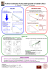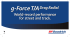### Hand Differences in Fanconi Anemia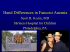### Cateterismo Cardíaco por vía Radial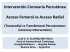### Spring 2011 Issue - Radial Engineering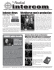### Problem Set 9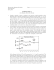### Dealer Application